x You are here: Home > Guides > Light Field Mapping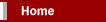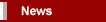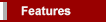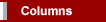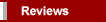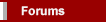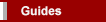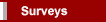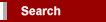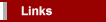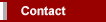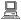xRobert Richmond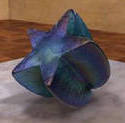Light Field Mapping

 September 2001 Page FourContents Page One: Applications of Light Field Mapping Synthetic Imaging Real-World Imaging Technical Analysis Page Four: Triangle Centered Approximation Triangle-Centered Light Field Mapping Analysis of Triangle-Centered Approximation Page Two: Data Acquisition Resampling and Normalization Page Five: Vertex-Centered Approximation Vertex-Centered Light Field Mapping Analysis of Vertex-Centered Approximation Page Three: Surface Light Field Approximation Page Six: Hardware Acceleration Light Field Map Compression Final ThoughtsTriangle Centered Approximation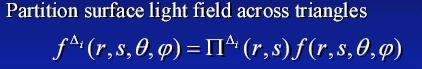One technique of approximation involves the usage of multiple triangles across the surface light field by defining a local reference frame for each partitioned triangular rendering area.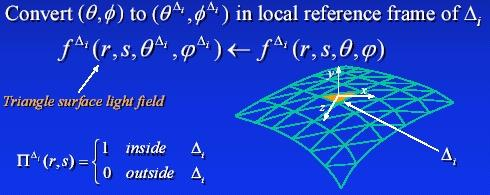A formula can now be constructed to represent the light map ranges for both within and outside the specific control area.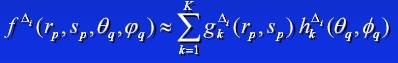Each triangular area is then decomposed independently so compression through truncation can be performed by the root mean square approximation of each light field.

Triangle-Centered Light Field Mapping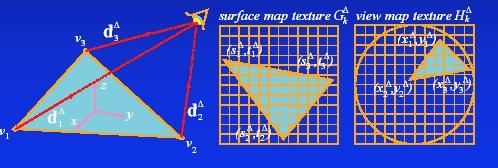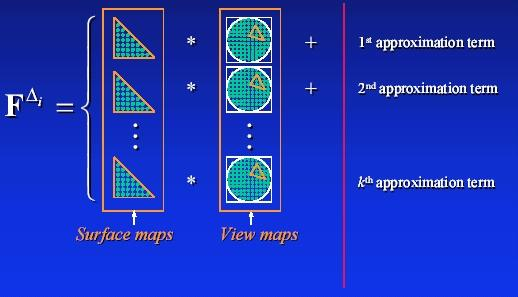Triangle-centered rendering produces the actual visual object by computing the local reference frame of each triangle using the pixel-per-pixel multiplication of both the surface and view map images.  The surface map data is static, while the view map data is dynamic according to the viewing direction.

Analysis of Triangle-Centered Approximation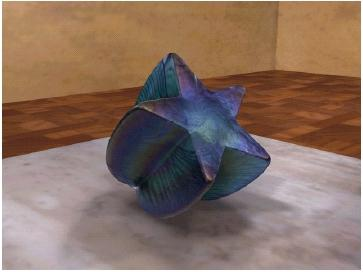Triangle-centered approximation has the advantage of being a relatively simple mathematical process, thus performance impact is kept within minimal boundaries.  However, this process does limit rendering accuracy, especially near the triangular edge layer boundaries.  Slight edge imperfections and discontinuities can be observed with the above image beyond the normal imaging issues present with JPEG compression.